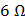# For what value of unknown resistancethe potential difference betweenandwill be zero in the circuit shown in the figurea)b)c)d)## Question ID - 150341 :- For what value of unknown resistancethe potential difference betweenandwill be zero in the circuit shown in the figurea)b)c)d)3537

 (b) For balanced Wheatstone bridgeNext Question :

Thegraph for a wire of copper of lengthand cross-section aresis shown in adjoining figure. The slope of the graph will bea) Less if the experiment is repeated at a higher temperature b) More if a wire of silver having the same dimension is used c) Doubled if the length of the wire is doubled d) Halved if length of the wire is halved